# Constraints

`semtree` allows different constraints on the split evaluation (`global.invariance`, `local.invariance`, and `focus.parameters`). These can be set in the following object and then passed to the `semtree` command:

``````library(semtree)

cnst <- semtree.constraints(local.invariance=NULL,
global.invariance=NULL,
focus.parameters=NULL)

semtree(model.x, data=df, constraints=cnst)
``````

## Simulation

To illustrate the invariance constraints, let's set up a latent factor model with four indicators and simulate some data with a known predictor structure. Let's create a dataset as follows:

• a latent construct, `lat`, measured by four indicators
• two predictors `p1` and `p2`
• only one predictor, `p1`, has an mean effect on the latent construct
• the other predictor, `p2`, has an effect on only one indicator (different loadings across groups; DIF)
``````N <- 2000
p1 <- sample(size=N, x=c(0,1), replace=TRUE)
p2 <- sample(size=N, x=c(0,1), replace=TRUE)
lat <- rnorm(N,mean = 0+p1)
observed[,3]<-observed[,3]+p2*0.5*lat
cfa.sim <- data.frame(observed,p1,p2)
names(cfa.sim)[1:4] <- paste0("x",1:4)
``````

Here is the OpenMx model specification

``````require("OpenMx");
manifests<-c("x1","x2","x3","x4")
latents<-c("F")
model.cfa <- mxModel("CFA", type="RAM", manifestVars = manifests,
latentVars = latents,
mxPath(from="F",to=c("x1","x2","x3","x4"),
free=c(TRUE,TRUE,TRUE,TRUE), value=c(1.0,1.0,1.0,1.0) ,
arrows=1, label=c("F__x1","F__x2","F__x3","F__x4") ),
mxPath(from="one",to=c("x2","x3","x4"),
free=c(TRUE,TRUE,TRUE), value=c(1.0,1.0,1.0) ,
arrows=1, label=c("const__x2","const__x3","const__x4") ),
mxPath(from="one",to=c("F"), free=c(TRUE),
value=c(1.0) , arrows=1, label=c("const__F") ),
mxPath(from="x1",to=c("x1"), free=c(TRUE),
value=c(1.0) , arrows=2, label=c("VAR_x1") ),
mxPath(from="x2",to=c("x2"), free=c(TRUE),
value=c(1.0) , arrows=2, label=c("VAR_x2") ),
mxPath(from="x3",to=c("x3"), free=c(TRUE),
value=c(1.0) , arrows=2, label=c("VAR_x3") ),
mxPath(from="x4",to=c("x4"), free=c(TRUE),
value=c(1.0) , arrows=2, label=c("VAR_x4") ),
mxPath(from="F",to=c("F"), free=c(FALSE),
value=c(1.0) , arrows=2, label=c("VAR_F") ),
mxPath(from="one",to=c("x1"), free=F, value=0, arrows=1),
mxData(cfa.sim, type = "raw")
);
``````

## Global Invariance

Global invariance is a relatively naive way of estimating a tree, in which a chosen set of parameters is equal across all leafs. This is achieved by fixing one or more selected parameters to the sample estimate before the tree is grown. Global invariance assures that the chosen parameters don't differ across the possible subgroups. However, if the parameters do differ, this leads to misspecification and jeopardizes the interpretation of the resulting tree structure. Using this approach is usually not recommended because it's typically not safe to assume that parameters are identical across a wide range of splits of the data. There may be cases in which it may make sense to constrain loading structures or means but it seems particularly dangerous to constrain variance components with such an approach.

``````tree.gc <- semtree(model.cfa, data=cfa.sim, constraints=
semtree.constraints(global.invariance =
c("F__x1","F__x2","F__x3","F__x4")))
#> Global Constraints:
#> F__x1 F__x2 F__x3 F__x4
#> Freely Estimated Parameters:
#> VAR_x1 VAR_x2 VAR_x3 VAR_x4 const__x2 const__x3 const__x4 const__F
``````
``````plot(tree.gc)
``````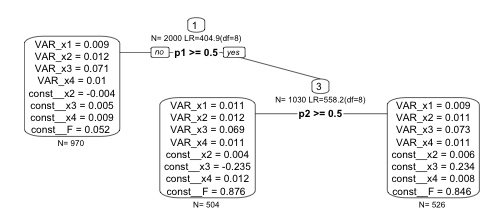Even though we retrieve both predictors in the tree, the tree structure does not represent the data generating model (`p2` is found to be conditional only on `p1`>=.5) and the misspecification in the loadings leads to bias in the mean structure.

## Local Invariance

Local invariance builds a tree under which all parameters across the leafs of a tree may differ but the chosen parameters may not differ significantly from each other. If they differed significantly, the respective split would not be considered as a valid split and would never be chosen. Local constraints are implemented by means of an additional test of measurement invariance. For each possible split, we fit an additional null model, in which the locally invariant parameters are constrained to be equal across the two resulting daugther nodes of a split. Only if we reject this null hypothesis, we believe that there is measurement non-invariance and disregard the split. A typical use-case is to the set of loadings of a factor model as `local.invariance` to allow a tree with weakly measurement-invariant leafs.

``````tree.lc <- semtree(model.cfa, data=cfa.sim, constraints=
semtree.constraints(
local.invariance= c("F__x1","F__x2","F__x3","F__x4")))
``````

Now we find `p1` as the only predictor that yields subgroups that pass the measurement invariance test. Even though we have chosen the four factor loadings as `local.invariance` constraint, they are allowed to differ numerically but there was no significant difference between them.

``````plot(tree.lc)
``````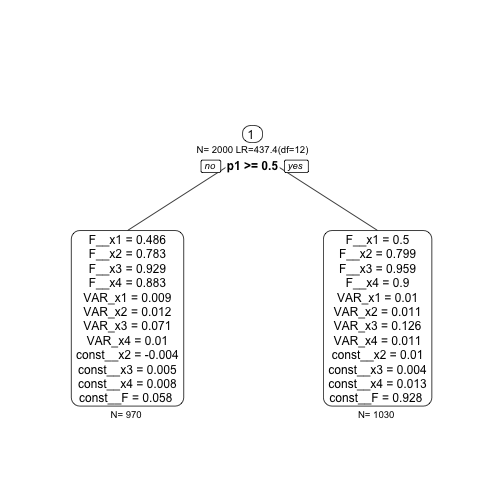## Focus Parameters

First, we create some data. Here, we generate 1,000 bivariate observations `x1` and `x2` gathered in a data frame called `obs`. Also, there are two predictors `grp1` and `grp2`. Predictor `grp1` predicts a mean difference in `x1` whereas `grp2` predicts differences only in `x2`.

``````set.seed(123)
N <- 1000
grp1 <- sample(x = c(0,1), size=N, replace=TRUE)
grp2 <- sample(x = c(0,1), size=N, replace=TRUE)
Sigma <- matrix(byrow=TRUE,
nrow=2,c(2,0.2,
0.2,1))
obs <- MASS::mvrnorm(N,mu=c(0,0),
Sigma=Sigma)
obs[,1] <- obs[,1] + ifelse(grp1,3,0)
obs[,2] <- obs[,2] + ifelse(grp2,3,0)
df.biv <- data.frame(obs, grp1, grp2)
names(df.biv)[1:2] <- paste0("x",1:2)
``````

A tree without constraints should recover both parameters (given large enough sample and effect sizes) because it explores differences in both means and covariance structure of `x1` and `x2`. Here is an OpenMx model specification for a saturated bivariate model:

``````manifests<-c("x1","x2")
model.biv <- mxModel("Bivariate_Model",
type="RAM",
manifestVars = manifests,
latentVars = c(),
mxPath(from="x1",to=c("x1","x2"),
free=c(TRUE,TRUE), value=c(1.0,.2) ,
arrows=2, label=c("VAR_x1","COV_x1_x2") ),
mxPath(from="x2",to=c("x2"), free=c(TRUE),
value=c(1.0) , arrows=2, label=c("VAR_x2") ),
mxPath(from="one",to=c("x1","x2"), label=c("mu1","mu2"),
free=TRUE, value=0, arrows=1),
mxData(df.biv, type = "raw")
);
``````

Let's run this model in OpenMx:

``````result <- mxRun(model.biv)
#> Running Bivariate_Model with 5 parameters
summary(result)
#> Summary of Bivariate_Model
#>
#> free parameters:
#>        name matrix row col  Estimate  Std.Error A
#> 1    VAR_x1      S   1   1 4.0283802 0.18015249
#> 2 COV_x1_x2      S   1   2 0.3039197 0.11443414
#> 3    VAR_x2      S   2   2 3.2282347 0.14437014
#> 4       mu1      M   1   1 1.4187340 0.06346921
#> 5       mu2      M   1   2 1.4628998 0.05681763
#>
#> Model Statistics:
#>                |  Parameters  |  Degrees of Freedom  |  Fit (-2lnL units)
#>        Model:              5                   1995              8233.926
#>    Saturated:              5                   1995                    NA
#> Independence:              4                   1996                    NA
#> Number of observations/statistics: 1000/2000
#>
#> Information Criteria:
#>       |  df Penalty  |  Parameters Penalty  |  Sample-Size Adjusted
#> AIC:       4243.926               8243.926                 8243.986
#> BIC:      -5547.046               8268.465                 8252.584
#> CFI: NA
#> TLI: 1   (also known as NNFI)
#> RMSEA:  0  [95% CI (NA, NA)]
#> Prob(RMSEA <= 0.05): NA
#> To get additional fit indices, see help(mxRefModels)
#> timestamp: 2020-01-07 11:01:14
#> Wall clock time: 0.01480794 secs
#> optimizer:  CSOLNP
#> OpenMx version number: 2.15.4
#> Need help?  See help(mxSummary)
``````

Now, we grow a tree without constraints:

``````
tree.biv <- semtree(model.biv, data=df.biv)
``````

As expected, we obtain a tree structure that has both `p1` and `p2`:

``````plot(tree.biv)
``````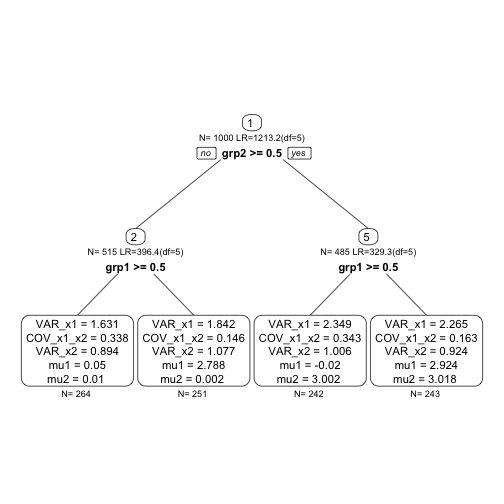Let us visualize what this tree is doing. Here is the empirical joint distribution of both `x1` and `x2`:

``````require("ggplot2")
ggplot(data = df.biv, aes(x=x1, y=x2))+
geom_density_2d()+
theme_classic()
``````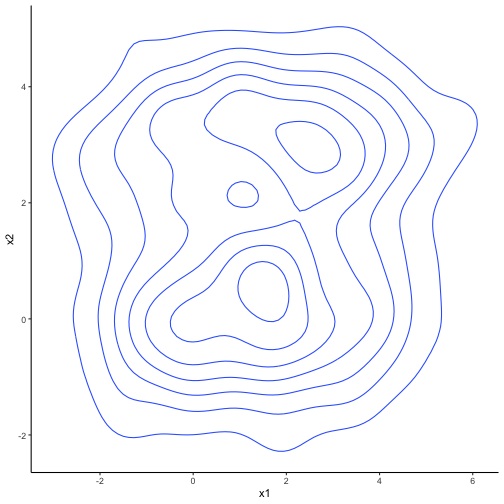And here is the partition of the observed two-dimensional space implied by the leafs of the tree:

``````df.biv.pred <- data.frame(df.biv,
leaf=factor(getLeafs(tree=tree.biv, data = df.biv)))
ggplot(data = df.biv.pred, aes(x=x1, y=x2))+
geom_density_2d(aes(colour=leaf))+
theme_classic()
``````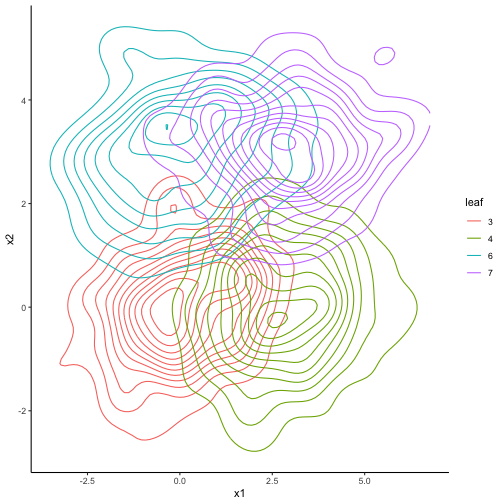What if we were interested only in splits with respect to one of the two dimensions? In this case, we can set a `focus.parameter`. Focus parameters change the split evaluation such that only splits are evaluated that maximize the misfit between a model in which all parameters are free and a model in which all parameters are free but the parameters given in `focus.parameter`.

Let us first set `mu1` as focus parameter:

``````
tree.biv2 <- semtree(model.biv, df.biv, constraints=
semtree.constraints(focus.parameters = "mu1"))

plot(tree.biv2)
``````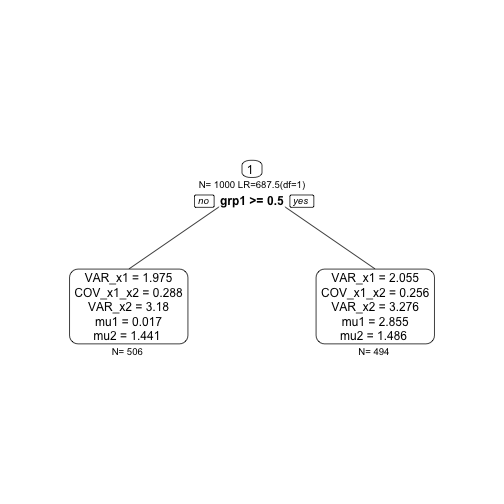As expected, the resulting tree structure only has `grp1` as predictor because `grp1` predicts differences in `mu1`. Predictor `grp2` did not come up anymore. Now, if we set `mu2`, we should see the exact opposite picture:

``````
tree.biv3 <- semtree(model.biv, df.biv, constraints=
semtree.constraints(focus.parameters = "mu2"))
``````

And, indeed, we see only `grp2` as predictor whereas `grp1` was not selected this time.

``````plot(tree.biv3)
``````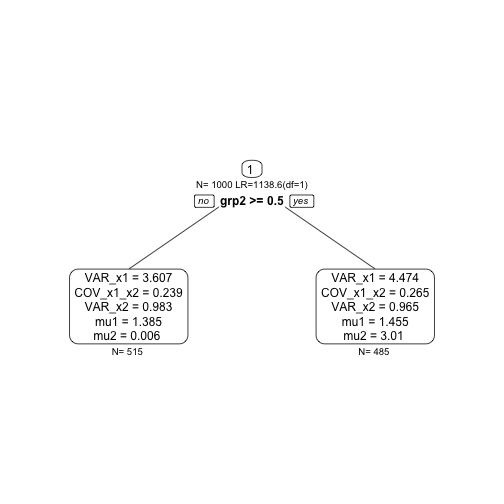Finally, we set the `focus.parameter` to one of the variance parameters. None of the predictors predicts differences in the variance, so we expect an empty tree (only the root node; no predictors selected):

``````
tree.biv4 <- semtree(model.biv, df.biv, constraints=
semtree.constraints(focus.parameters = "VAR_x2"))

plot(tree.biv4)
``````